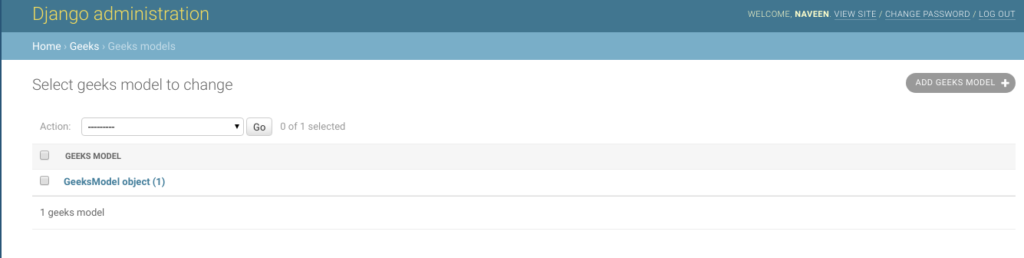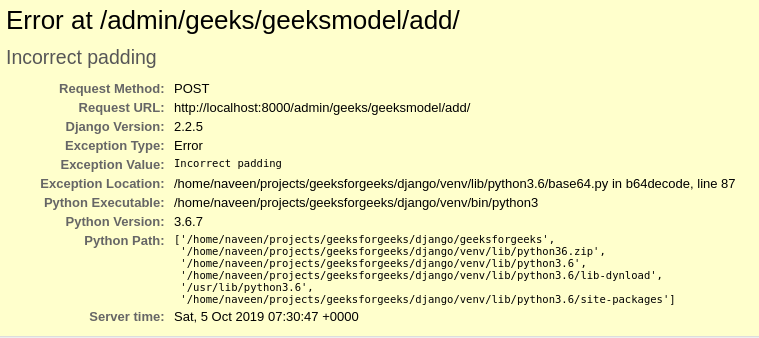Open in App
Not now

# BinaryField – Django Models

• Difficulty Level : Basic
• Last Updated : 05 Aug, 2021

BinaryField is a special field to store raw binary data. It can be assigned bytes, bytearray, or memoryview. By default, BinaryField sets editable to False, that is it can’t be included in a ModelForm. Since BinaryField stores raw data or in other terms a python object, it can not be manually entered and needs to be assigned through a view or django shell. This is what editable to False means, that is it can’t be edited through any form.

Syntax

`field_name = models.BinaryField(**options)`

## Django Model BinaryField Explanation

Illustration of BinaryField using an Example. Consider a project named geeksforgeeks having an app named geeks.

Refer to the following articles to check how to create a project and an app in Django.

Enter the following code into models.py file of geeks app.

## Python3

 `from` `django.db ``import` `models``from` `django.db.models ``import` `Model``# Create your models here.` `class` `GeeksModel(Model):``    ``geeks_field ``=` `models.BinaryField()`

Add the geeks app to INSTALLED_APPS

## Python3

 `# Application definition` `INSTALLED_APPS ``=` `[``    ``'django.contrib.admin'``,``    ``'django.contrib.auth'``,``    ``'django.contrib.contenttypes'``,``    ``'django.contrib.sessions'``,``    ``'django.contrib.messages'``,``    ``'django.contrib.staticfiles'``,``    ``'geeks'``,``]`

Now when we run makemigrations command from the terminal,

`Python manage.py makemigrations`

A new folder named migrations would be created in geeks directory with a file named 0001_initial.py

## Python3

 `# Generated by Django 2.2.5 on 2019-09-25 06:00` `from` `django.db ``import` `migrations, models` `class` `Migration(migrations.Migration):` `    ``initial ``=` `True` `    ``dependencies ``=` `[``    ``]` `    ``operations ``=` `[``        ``migrations.CreateModel(``            ``name ``=``'GeeksModel'``,``            ``fields ``=``[``                ``(``'id'``,``                  ``models.AutoField(auto_created ``=` `True``,``                  ``primary_key ``=` `True``,``                  ``serialize ``=` `False``,``                  ``verbose_name ``=``'ID'``                ``)),``                ``(``'geeks_field'``, models.BinaryField()),``            ``],``        ``),``    ``]`

Thus, an geeks_field BigIntegerField is created when you run makemigrations on the project.It is a field to store raw binary data.

## How to use BinaryField ?

Any type of data can be converted into bytes including integers, strings, images, etc. Let’s try to save a string into the BinaryField. String can be converted to bytes using the generic bytes function. This function internally points to CPython Library which implicitly calls the encode function for converting the string to the specified encoding.

## Python3

 `# importing the model``# from geeks app``from` `geeks.models ``import` `GeeksModel` `# creating a string``test_string ``=` `"GFG is best"` `# creating a bytes object``res ``=` `bytes(test_string, ``'utf-8'``)` `# creating a instance of``# GeeksModel``geek_object ``=` `GeeksModel.objects.create(geeks_field ``=` `res)``geek_object.save()`

Now let’s check it in admin server. We have created an instance of GeeksModelPossible errors
As specified, BinaryField is a non-editable field, if you manually try to make it editable using editable=True it will create unexpected errors. It should be created in a function so that objects can be allocated to it.## Field Options

Field Options are the arguments given to each field for applying some constraint or imparting a particular characteristic to a particular Field. For example, adding an argument null = True to BinaryField will enable it to store empty values for that table in relational database.

Here are the option and attributes that an BinaryField can use.

My Personal Notes arrow_drop_up